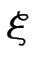## 9.4.11 Vectors

A variable representing a vector can be typeset using the command:

\vec{<variable>}

Example:Vectors are often typeset in bold. This can be done by redefining the \vec command. You could use \mathbf, for example:However, as you may have noticed, the Greek letterhas not come out in bold. Here's an alternative (using \boldsymbol defined in the amsfonts package):Located (or position) vectors, on the other hand, are usually typeset with a right arrow, but the default definition of \vec produces an arrow that is too small:Instead, use \overrightarrow (Table 9.10):You might prefer to define separate commands for a located vector and a vector variable.

Example:

In the preamble, define \lvec for a located vector and \bvec for a vector variable:

Later in the document:

Let $\bvec{u}=(x, y)$ represent $\lvec{OP}$, then
$\lVert \bvec{u} \rVert = \sqrt{x^2 + y^2}$Exercise 23: Maths: Vectors and Arrays

Try to produce the following: